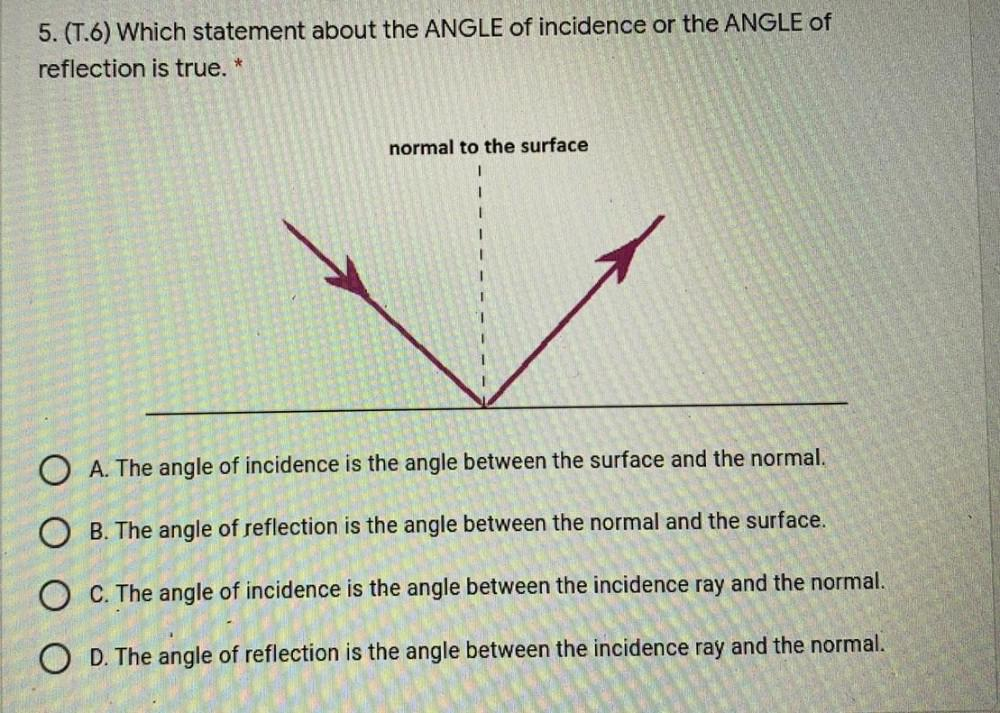Question:

# 5. (T.6) Which statement about the ANGLE of incidence or the ANGLE of reflection is true. * normal to the surface O A. The angle5. (T.6) Which statement about the ANGLE of incidence or the ANGLE of reflection is true. * normal to the surface O A. The angle of incidence is the angle between the surface and the normal. O B. The angle of reflection is the angle between the normal and the surface. O C. The angle of incidence is the angle between the incidence ray and the normal. O D. The angle of reflection is the angle between the incidence ray and the normal.